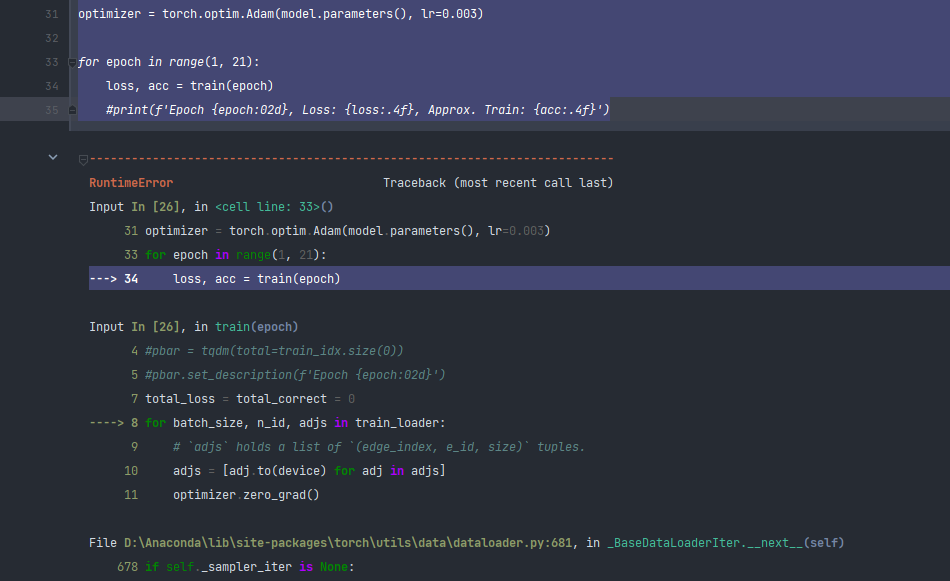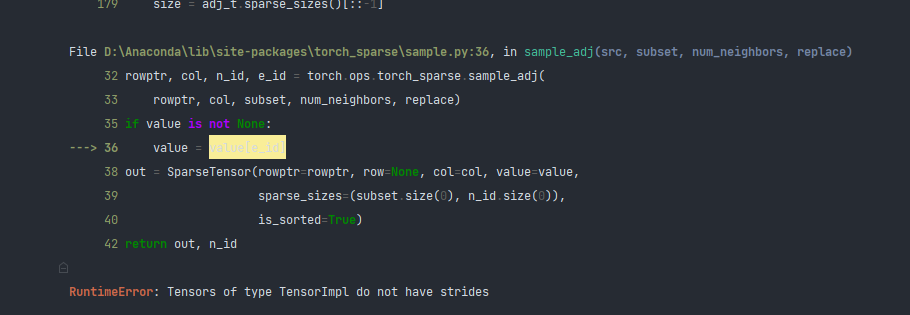Lukas00990 2022-09-19 20:02 采纳率: 41.8%

# RuntimeError: Tensors of type TensorImpl do not have strides``````from torch.utils.data import DataLoader, Dataset
import torch_geometric
import torch
import torch.nn.functional as F
from tqdm import tqdm
from torch_geometric.data import NeighborSampler
from torch_geometric.nn import SAGEConv
import os.path as osp
import pandas as pd
import numpy as np
import collections
from pandas.core.common import flatten
# importing obg datatset
from ogb.nodeproppred import PygNodePropPredDataset, Evaluator
from pandas.core.common import flatten
import seaborn as sns
import matplotlib.pyplot as plt
sns.set(rc={'figure.figsize':(16.7,8.27)})
sns.set_theme(style="ticks")
import collections
from scipy.special import softmax
import umap

root = osp.join(osp.dirname(osp.realpath('./')), 'data', 'products', 'ogbn-products')
dataset = PygNodePropPredDataset('ogbn-products', root)

# split_idx contains a dictionary of train, validation and test node indices
split_idx = dataset.get_idx_split()
# predefined ogb evaluator method used for validation of predictions
evaluator = Evaluator(name='ogbn-products')

# lets check the node ids distribution of train, test and val
print('Number of training nodes:', split_idx['train'].size(0))
print('Number of validation nodes:', split_idx['valid'].size(0))
print('Number of test nodes:', split_idx['test'].size(0))

data = dataset

# lets check some graph statistics of ogb-product graph
print("Number of nodes in the graph:", data.num_nodes)
print("Number of edges in the graph:", data.num_edges)
print("Node feature matrix with shape:", data.x.shape) # [num_nodes, num_node_features]
print("Graph connectivity in COO format with shape:", data.edge_index.shape) # [2, num_edges]
print("Target to train against :", data.y.shape)
print("Node feature length", dataset.num_features)
#%%
# checking the number of unique labels
# there are 47 unique categories of product
data.y.unique()
# load integer to real product category from label mapping provided inside the dataset
# lets see some of the product categories

# creating a dictionary of product category and corresponding integer label
label_idx, prod_cat = df.iloc[: ,0].values, df.iloc[: ,1].values
label_mapping = dict(zip(label_idx, prod_cat))
# counting the numbers of samples for each category
y = data.y.tolist()
y = list(flatten(y))
count_y = collections.Counter(y)
print(count_y)
df[:10]

train_idx = split_idx['train']
#                               sizes=[15, 10, 5], batch_size=1024,
#                               shuffle=True)

node_idx=train_idx, sizes=[15, 10, 5],
batch_size=1024, shuffle=True)

class SAGE(torch.nn.Module):
def __init__(self, in_channels, hidden_channels, out_channels, num_layers=3):
super(SAGE, self).__init__()

self.num_layers = num_layers

self.convs = torch.nn.ModuleList()
self.convs.append(SAGEConv(in_channels, hidden_channels))
for _ in range(num_layers - 2):
self.convs.append(SAGEConv(hidden_channels, hidden_channels))
self.convs.append(SAGEConv(hidden_channels, out_channels))

def reset_parameters(self):
for conv in self.convs:
conv.reset_parameters()

# `train_loader` computes the k-hop neighborhood of a batch of nodes,
# and returns, for each layer, a bipartite graph object, holding the
# bipartite edges `edge_index`, the index `e_id` of the original edges,
# and the size/shape `size` of the bipartite graph.
# Target nodes are also included in the source nodes so that one can
# easily apply skip-connections or add self-loops.
for i, (edge_index, _, size) in enumerate(adjs):
xs = []
x_target = x[:size]  # Target nodes are always placed first.
x = self.convs[i]((x, x_target), edge_index)
if i != self.num_layers - 1:
x = F.relu(x)
x = F.dropout(x, p=0.5, training=self.training)
xs.append(x)
if i == 0:
x_all = torch.cat(xs, dim=0)
layer_1_embeddings = x_all
elif i == 1:
x_all = torch.cat(xs, dim=0)
layer_2_embeddings = x_all
elif i == 2:
x_all = torch.cat(xs, dim=0)
layer_3_embeddings = x_all
#return x.log_softmax(dim=-1)
return layer_1_embeddings, layer_2_embeddings, layer_3_embeddings

def inference(self, x_all):
pbar = tqdm(total=x_all.size(0) * self.num_layers)
pbar.set_description('Evaluating')

# Compute representations of nodes layer by layer, using *all*
# available edges. This leads to faster computation in contrast to
# immediately computing the final representations of each batch.
total_edges = 0
for i in range(self.num_layers):
xs = []
total_edges += edge_index.size(1)
x = x_all[n_id].to(device)
x_target = x[:size]
x = self.convs[i]((x, x_target), edge_index)
if i != self.num_layers - 1:
x = F.relu(x)
xs.append(x)

pbar.update(batch_size)

if i == 0:
x_all = torch.cat(xs, dim=0)
layer_1_embeddings = x_all
elif i == 1:
x_all = torch.cat(xs, dim=0)
layer_2_embeddings = x_all
elif i == 2:
x_all = torch.cat(xs, dim=0)
layer_3_embeddings = x_all

pbar.close()

return layer_1_embeddings, layer_2_embeddings, layer_3_embeddings

device = torch.device('cuda' if torch.cuda.is_available() else 'cpu')
model = SAGE(dataset.num_features, 256, dataset.num_classes, num_layers=3)
model = model.to(device)
x = data.x.to(device)
y = data.y.squeeze().to(device)

def train(epoch):
model.train()

#pbar = tqdm(total=train_idx.size(0))
#pbar.set_description(f'Epoch {epoch:02d}')

total_loss = total_correct = 0
# `adjs` holds a list of `(edge_index, e_id, size)` tuples.
l1_emb, l2_emb, l3_emb = model(x[n_id], adjs)
#print("Layer 1 embeddings", l1_emb.shape)
#print("Layer 2 embeddings", l1_emb.shape)
out = l3_emb.log_softmax(dim=-1)
loss = F.nll_loss(out, y[n_id[:batch_size]])
loss.backward()
optimizer.step()

total_loss += float(loss)
total_correct += int(out.argmax(dim=-1).eq(y[n_id[:batch_size]]).sum())
#pbar.update(batch_size)

#pbar.close()

approx_acc = total_correct / train_idx.size(0)

return loss, approx_acc

for epoch in range(1, 21):
loss, acc = train(epoch)
#print(f'Epoch {epoch:02d}, Loss: {loss:.4f}, Approx. Train: {acc:.4f}')
``````• 写回答

#### 悬赏问题

• ¥15 求一个超难的动态新增元素的 click 事件无效的解决办法。
• ¥20 怎么修改mediawiki允许上传的文件大小？
• ¥15 agrySEXPAYm 是毒吗
• ¥50 Java实现注册登录实现数据库增删改查功能,数据库至少两个表
• ¥20 求解R语言的数据分析问题
• ¥20 求GD32F105和305解除读保护方法教程
• ¥15 C++代码优化，复杂度太高，无法通过clang-tidy检查，用什么办法可以优=化
• ¥15 关于跨链隐私保护方案
• ¥15 node mongodb 根据id给子集合list添加对象 请问应该如何操作
• ¥50 如何得到路径下的绝对路径并且回传到list上